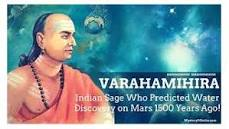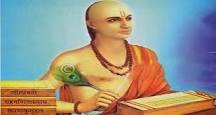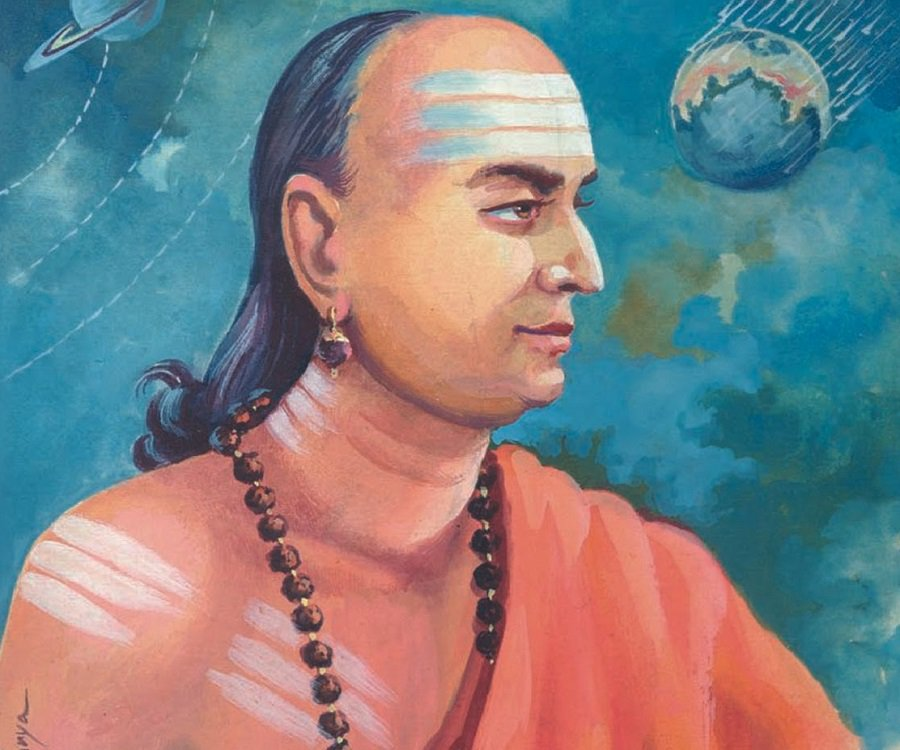# RGUKT### Biography of Varahamihira

Born : 505 CE,Ujjain. Died : 587 CE. Education : Astronomer,Mathematician. Period : Gupta Era Works :

1) He improved the accuracy of sine tables of aryabhatta. 2) He defined the algebraic properties of zero as well as negative numbers. 3) He was among the first mathematician to discover a version of what is now known as the pascal’s triang### Biography of BrahmaGupta

Born : 598 AD, Bhinmal in Rajhasthan

Works : * Brahmagupta was the first to give rules to compute with zero

* Brahmagupta gave the solution of the general linear equation as bx + c = dx + e equivalent to x = e − c/b − d

* Brahmagupta then goes on to give the sum of the squares and cubes of the first n integers sum of the squares of the first n natural numbers as n(n + 1)(2n + 1)/6 sum of the cubes of the first n natural numbers as (n(n + 1)/2)^2 * Brahmagupta states that 0/0 is undefined * He described about addition, subtractioBorn in Bijapur in Karnataka Works : * Sidhanta shiromani(“crown of Treatises”in Sanskrit)

1. Lilavathi (name of his daughter) * It consists of 277 verses * It is also known as Patiganita or ankaganita which deals with the calculations,progressions,permutations 2. Bijaganita * It consists of 213 verses * It discusses zero and infinity(that any number divided by zero gives infinity and the formula like,sin(A+-B)=sinA.cosB+-cosAsinB

3. Goladhyaya * It deals with spheres( The hundredth part of a circle seems### Aryabhata

Aryabhata

Born : 476 CE in Kusumapura, pataliputra (present day Patna). Died : 550 CE

Works : * Place value system and zero

* Approximation of pi He concluded that pi is irrational which is accurate to five significant figure 3.1416

* He gave the area of triangle as “for a triangle, the result of a side of a perpendicular with the half side is the area”

* He discussed the concept of sine * Indeterminant equations *Aryabhatta provided elegant results for the summation of series of squares and cubes 1^2+2^2+......+n^2=n(n